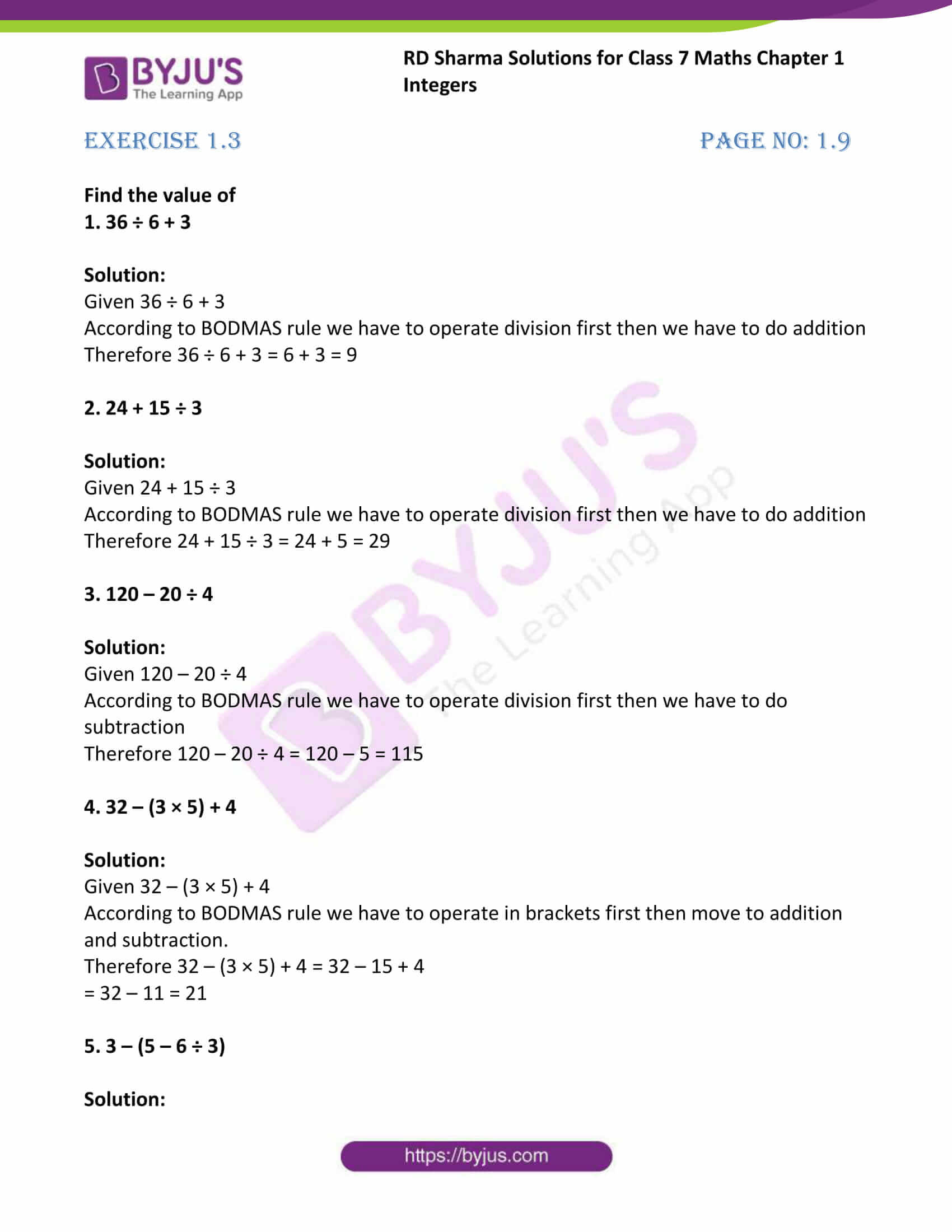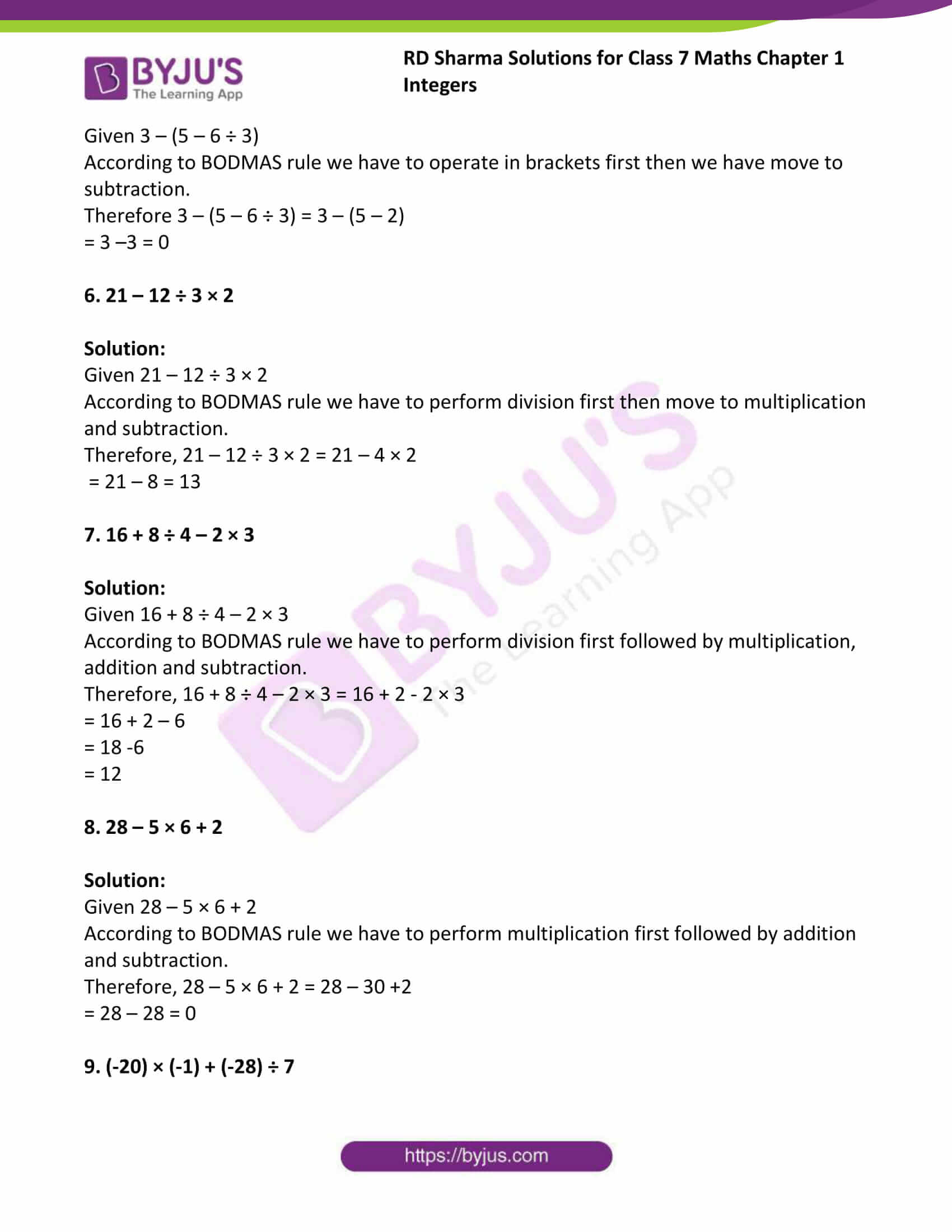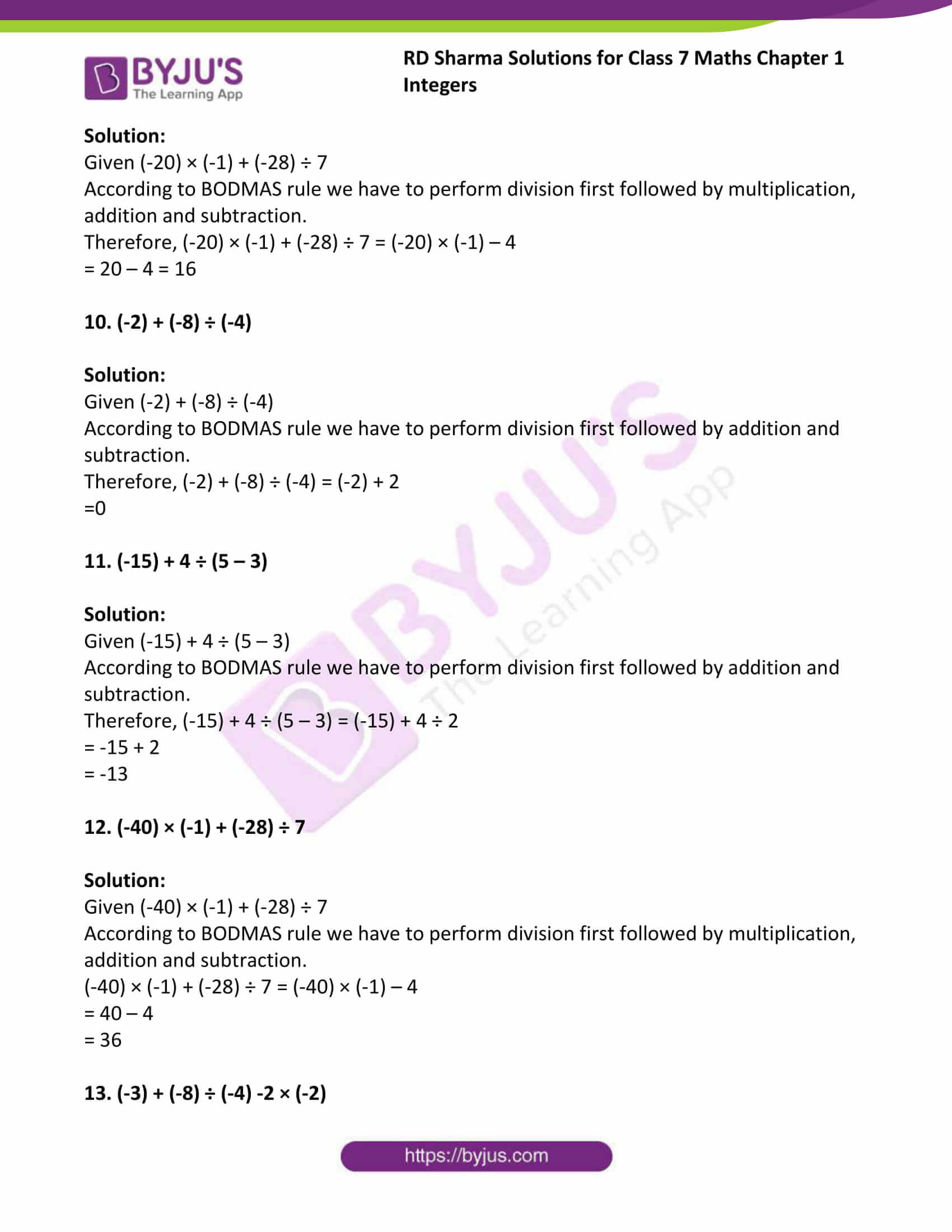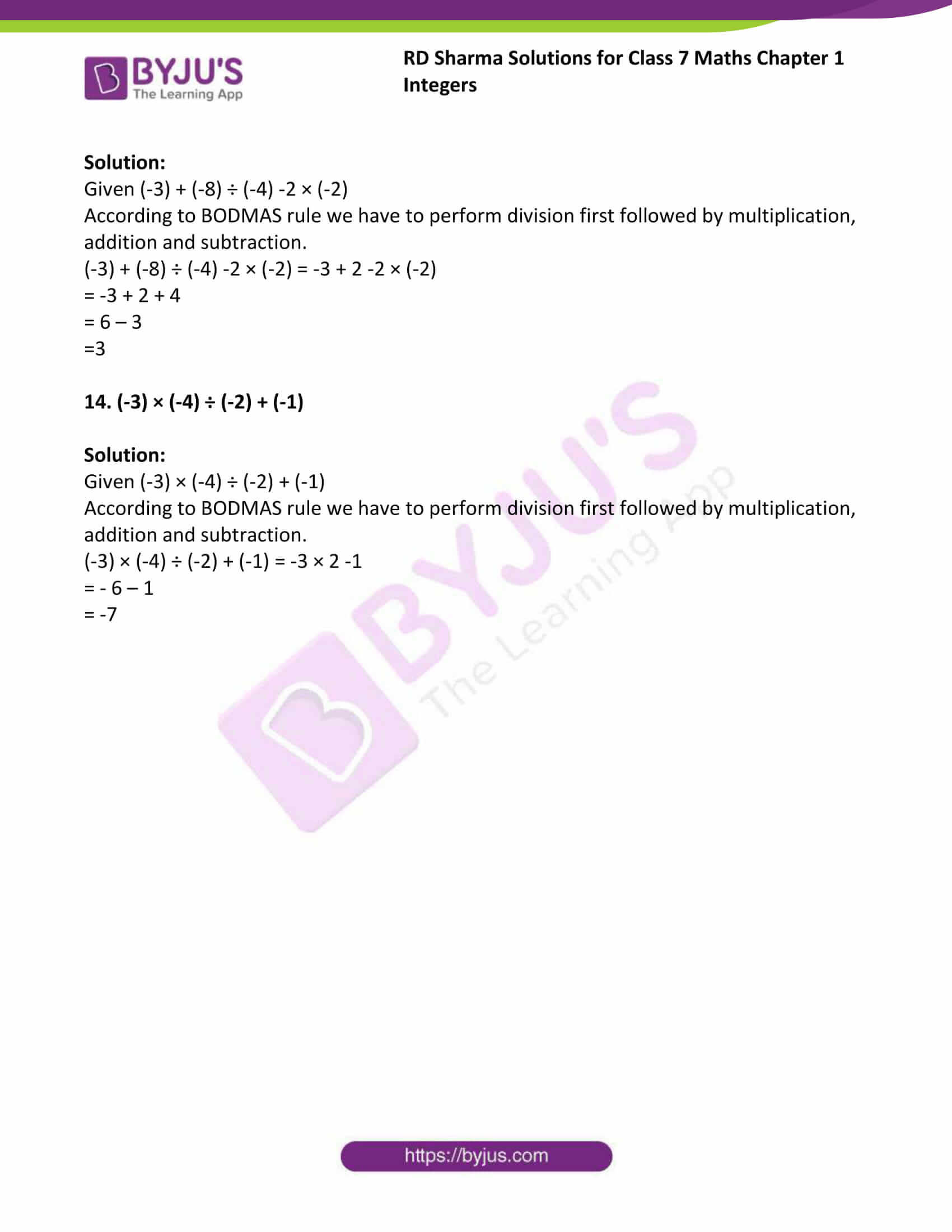# RD Sharma Solutions For Class 7 Maths Chapter 1 Integers Exercise 1.3

RD Sharma Solutions for Class 7 Maths Chapter 1 Exercise 1.3 are provided here. The questions present in this exercise have been solved by BYJU’S subject experts, and this will help students in solving the questions without any obstacles. The solutions prepared are according to the RD Sharma Solutions guidelines and ensure that each and every concept is understood. This exercise includes the precedence of operations of addition, subtraction and multiplication. By practising the RD Sharma Solutions for Class 7, students can excel in the board exams.

## Download the PDF of RD Sharma Solutions For Class 7 Maths Chapter 1 – Integers Exercise 1.3### Access answers to Maths RD Sharma Solutions For Class 7 Chapter 1 – Integers Exercise 1.3

Exercise 1.3 Page No: 1.9

Find the value of

1. 36 ÷ 6 + 3

Solution:

Given 36 ÷ 6 + 3

According to BODMAS rule we have to operate division first then we have to do addition

Therefore 36 ÷ 6 + 3 = 6 + 3 = 9

2. 24 + 15 ÷ 3

Solution:

Given 24 + 15 ÷ 3

According to BODMAS rule we have to operate division first then we have to do addition

Therefore 24 + 15 ÷ 3 = 24 + 5 = 29

3. 120 – 20 ÷ 4

Solution:

Given 120 – 20 ÷ 4

According to BODMAS rule we have to operate division first then we have to do subtraction

Therefore 120 – 20 ÷ 4 = 120 – 5 = 115

4. 32 – (3 × 5) + 4

Solution:

Given 32 – (3 × 5) + 4

According to BODMAS rule we have to operate in brackets first then move to addition and subtraction.

Therefore 32 – (3 × 5) + 4 = 32 – 15 + 4

= 32 – 11 = 21

5. 3 – (5 – 6 ÷ 3)

Solution:

Given 3 – (5 – 6 ÷ 3)

According to BODMAS rule we have to operate in brackets first then we have move to subtraction.

Therefore 3 – (5 – 6 ÷ 3) = 3 – (5 – 2)

= 3 –3 = 0

6. 21 – 12 ÷ 3 × 2

Solution:

Given 21 – 12 ÷ 3 × 2

According to BODMAS rule we have to perform division first then move to multiplication and subtraction.

Therefore, 21 – 12 ÷ 3 × 2 = 21 – 4 × 2

= 21 – 8 = 13

7. 16 + 8 ÷ 4 – 2 × 3

Solution:

Given 16 + 8 ÷ 4 – 2 × 3

According to BODMAS rule we have to perform division first followed by multiplication, addition and subtraction.

Therefore, 16 + 8 ÷ 4 – 2 × 3 = 16 + 2 – 2 × 3

= 16 + 2 – 6

= 18 -6

= 12

8. 28 – 5 × 6 + 2

Solution:

Given 28 – 5 × 6 + 2

According to BODMAS rule we have to perform multiplication first followed by addition and subtraction.

Therefore, 28 – 5 × 6 + 2 = 28 – 30 +2

= 28 – 28 = 0

9. (-20) × (-1) + (-28) ÷ 7

Solution:

Given (-20) × (-1) + (-28) ÷ 7

According to BODMAS rule we have to perform division first followed by multiplication, addition and subtraction.

Therefore, (-20) × (-1) + (-28) ÷ 7 = (-20) × (-1) – 4

= 20 – 4 = 16

10. (-2) + (-8) ÷ (-4)

Solution:

Given (-2) + (-8) ÷ (-4)

According to BODMAS rule we have to perform division first followed by addition and subtraction.

Therefore, (-2) + (-8) ÷ (-4) = (-2) + 2

=0

11. (-15) + 4 ÷ (5 – 3)

Solution:

Given (-15) + 4 ÷ (5 – 3)

According to BODMAS rule we have to perform division first followed by addition and subtraction.

Therefore, (-15) + 4 ÷ (5 – 3) = (-15) + 4 ÷ 2

= -15 + 2

= -13

12. (-40) × (-1) + (-28) ÷ 7

Solution:

Given (-40) × (-1) + (-28) ÷ 7

According to BODMAS rule we have to perform division first followed by multiplication, addition and subtraction.

(-40) × (-1) + (-28) ÷ 7 = (-40) × (-1) – 4

= 40 – 4

= 36

13. (-3) + (-8) ÷ (-4) -2 × (-2)

Solution:

Given (-3) + (-8) ÷ (-4) -2 × (-2)

According to BODMAS rule we have to perform division first followed by multiplication, addition and subtraction.

(-3) + (-8) ÷ (-4) -2 × (-2) = -3 + 2 -2 × (-2)

= -3 + 2 + 4

= 6 – 3

=3

14. (-3) × (-4) ÷ (-2) + (-1)

Solution:

Given (-3) × (-4) ÷ (-2) + (-1)

According to BODMAS rule we have to perform division first followed by multiplication, addition and subtraction.

(-3) × (-4) ÷ (-2) + (-1) = -3 × 2 -1

= – 6 – 1

= -7

## RD Sharma Solutions for Class 7 Maths Chapter – 1 Integers Exercise 1.3

Exercise 1.3 of RD Sharma Solutions for Chapter 1, Integers explains the operator precedence. Here students will get to know about the BODMAS rule, which is essential in higher studies as well as in daily life. Here the simplification of the mathematical equations or expressions consisting of the same type of operation explains about the operator precedence.